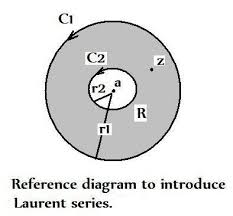## Tuesday, December 17, 2019

### Revisiting Laurent Series

We introduce the Laurent Series as follows:

If f(z) is analytic inside and on the boundary of the ring-shaped region R (see diagram) bounded by two concentric circles C1 and C2 with center at a and respective radii r1 and r2 (r1 > r2) then for all z in R:f(z) = å¥ n = -¥   c n (z – a) n  +    å¥ n =-¥   c -n /   (z – a) n

where:

c n  =  1/2 pò C1  f(w) dw /(w – a) n+1            n = 0,1, 2…..

c - n  =  1/2 pò C2  f(w) dw /(w – a) –n +1            n = -1,-2, -3…..

The other part of the series, consisting of negative powers, is called the principal part.  Either part or both may terminate or be identically zero. If the principal part is identically zero then f(z) is analytic at z = a since the derivative exists and the Laurent series is identical to the Taylor series.

More intricacies – and singularities:

Point z = a is called a zero or root of the function f(z) if f(a) = 0. If then f(z) is analytic at at z = a then the Taylor series:

f(z) = å¥ n = 0   c n (z – a) n

must have  c 0  = 0.  If c 1  ¹ 0, the point a is called a simple zero (or a zero of order one). It could happen that c 1 and perhaps several other next coefficients vanish. Then let  c m be the next vanishing coefficient (unless f(z) = 0) then the zero is said to be of order m. The order of a zero may be evaluated – without any knowledge of the Taylor series – by calculating:

lim z® a      f(z)  / (z – a) n

For n = 1, 2, 3. The lowest value of n for which this limit doesn’t vanish is equal to the order of the zero.

If a function f(z) is analytic in the neighborhood of some point z = a with the exception of the point z = a itself then it is said to have an isolated singularity (or isolated singular point) at z = a. It’s customary to distinguish isolated singularities by the following types of behavior of f(z) as z  ® a for an arbitrary function.

Examples:

1)     f(z)  remains bounded, i.e. ÷ f(z)÷  <B for a fixed B

2)     f(z) is not bounded and ÷ f(z)÷    approaches infinity. Namely,

÷ f(z)÷   > M for ÷ z – a ÷  <  e

3)     Neither of the two cases above, in other words f(z) oscillates.

Examples:
1)     f(z) =  1/ z – 1  (isolated singularity at z = 1)

a) If we demand ÷ z ÷  < 1 we can obtain a Taylor expansion.

b) ) If we seek:  1 < ÷ z ÷   <   0    we obtain a Laurent expansion

2)     f(z) = 1/ z (1 – z)

a) If  0 <  ÷ z ÷   <   1  then we obtain a Laurent series

b)  If   1   < ÷ z ÷   <   0    we also obtain a Laurent expansion.

Problem for the Math Maven

Consider the function: f(z) = 1/ (z+ 1) ((z + 3)

a) Find a  Laurent series  for:   1< ÷ z ÷   < 3

b) Find a Laurent series for    0 ÷ z +  1 ÷  < 2